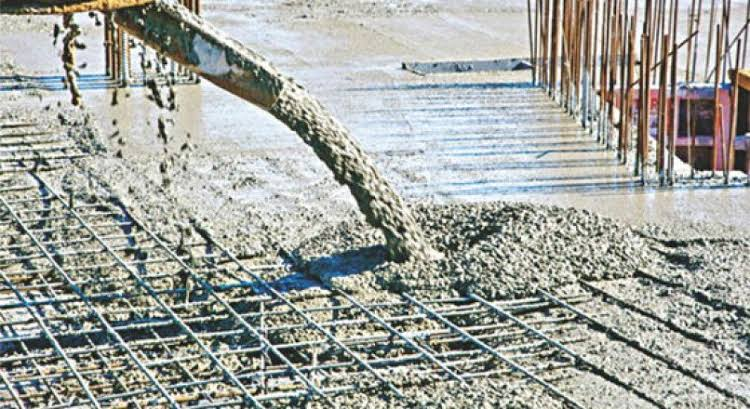# Cement Concrete Calculator  IS 456

##Cement Concrete Calculation

meter
cm
meter
cm
meter
cm

#### Total Volume of Cement Concrete

280.00 m3
9888.12 ft3
Sr. Material Quantity
1Cement 2216.73 Bags
2Sand 180.39 Ton
3Aggregate 314.22 Ton

## Cement Concrete Calculation

#### Cement Concrete Volume

$=\mathrm{Length}×\mathrm{Width}×\mathrm{Depth}$

$=\mathrm{10.00}×\mathrm{7.00}×\mathrm{4.00}$

#### Wet Volume of Mix

$=\mathrm{Total Volume}+\left(\mathrm{Total Volume}×\frac{52.4}{100}\right)$

$=\mathrm{280.00}+\left(\mathrm{280.00}×\frac{52.4}{100}\right)$

Wet volume of mix is 52.4 % higher than dry volume

#### Cement Volume

$=\frac{Cement Ratio}{\mathrm{Sum of Ratio}}×\mathrm{Wet Volume of Mix}$

$=\frac{1}{\mathrm{5.5}}×\mathrm{426.72}$

#### No. of Cement Bags

$=\frac{\mathrm{Cement Volume}}{0.035}$

$=\frac{\mathrm{77.59}}{0.035}$

#### Cement in Kg

$=\mathrm{No. of Cement Bags}×50$

$=\mathrm{2216.73}×50$

Note: 1 Bag of cement = 0.035 m3.
1 Cement bag contains = 50 kg cement

#### Sand Volume

$=\frac{Sand Ratio}{\mathrm{Sum of Ratio}}×\mathrm{Wet Volume of Mix}$

$=\frac{1.5}{\mathrm{5.5}}×\mathrm{426.72}$

#### Sand in Kg

$=\mathrm{Sand Volume}×1550$

$=\mathrm{116.38}×1550$

#### Sand in Ton

$=\frac{\mathrm{Sand in Kg.}}{1000}$

$=\frac{\mathrm{180386.18}}{1000}$

Note: By considering dry loose bulk density of sand = 1550 kg/m3.
1000 kg = 1 Ton

#### Aggregate Volume

$=\frac{Aggregate Ratio}{\mathrm{Sum of Ratio}}×\mathrm{Wet Volume of Mix}$

$=\frac{3}{\mathrm{5.5}}×\mathrm{426.72}$

#### Aggregate in Kg

$=\mathrm{Aggregate Volume}×1350$

$=\mathrm{232.76}×1350$

#### Aggregate in Ton

$=\frac{\mathrm{Aggregates in Kg.}}{1000}$

$=\frac{\mathrm{314221.09}}{1000}$

Note: By considering dry loose bulk density of aggregate = 1350 kg/m3.
1000 kg = 1 Ton

## What is PCC (Plain Cement Concrete) Calculation?

The term PCC stands for plain cement concrete. The mixture of cement, fine aggregate (sand) and coarse aggregate are generally called plain cement concrete (PCC).

Plain Cement Concrete (PCC) is also called as Cement Concrete (CC) or Blinding Concrete. It is used for leveling, bedding for footings, grade slabs, concrete roads etc. PCC is used to provide non-porous, rigid, impervious, firm and leveled bed for laying RCC, where earth is soft and yielding. PCC can be use over brick flat soling or without brick flat soling. PCC also used as filler like lump concrete; this is a mix of PCC and boulder. It consists of cement, sand and coarse aggregates mixed with water in the specified proportions.Concrete code and Concrete ratio:
Concrete Grade Proportion of Cement : Sand : Stone pieces Expected Compressive Strength at 28 days
M10 or M100 1 : 3 : 6 10 N/mm2 or 100 Kg/cm2
M20 or M200 1 : 2 : 4 15 N/mm2 or 150 Kg/cm2
M20 or M200 1 : 1.5 : 3 20 N/mm2 or 200 Kg/cm2
M25 or M250 1 : 1 : 2 25 N/mm2 or 250 Kg/cm2

## Strength of PCC (Plain Cement Concrete)/Nominal Mix:

Strength of PCC is defined as compressive strength after 28 days, expressed as M15, M20, where M stands for Mix and 15 stands for 15 N/mm2 (n/mm2 must be read as 'Newton’s per millimeter Cubic') compressive strength at 28 days. The proportions of materials (cement, sand, coarse aggregate) for nominal mix/design mix concrete that are normally used are 1:3:6 or 1:4:8. As strength is not a major criterion, the design mix is generally avoided.

## What is RCC calculation?

The term RCC stands for Reinforced cement concrete. The mixture of cement, fine aggregate (sand) and coarse aggregate and reinforcement to increase its compressive and tensile strength are generally called Reinforced cement concrete (RCC).

Reinforced cement concrete or RCC is a composite material in which concrete's relatively low tensile strength and ductility are counteracted by the inclusion of reinforcement having higher tensile strength or ductility. The reinforcement is usually, though not necessarily, steel reinforcing bars (rebar) and is usually embedded passively in the concrete before the concrete sets. Reinforcing schemes are generally designed to resist tensile stresses in particular regions of the concrete that might cause unacceptable cracking and/or structural failure. Modern reinforced concrete can contain varied reinforcing materials made of steel, polymers or alternate composite material in conjunction with rebar or not. Reinforced concrete may also be permanently stressed (concrete in compression, reinforcement in tension), so as to improve the behavior of the final structure under working loads.

##### A lot of variety exists in RCC and there are different types of RCC:
• Footing (Pedastal footing, Raft footing, slop footing)
• Column
• Beam
• Slab
• Lintle## Strength of RCC/nominal mix:

Strength of RCC is defined as compressive strength after 28 days, expressed as M15, M20, where M stands for Mix and 15 stands for 15 N/mm2 (n/mm2 must be read as 'Newton’s per millimeter Cubed) compressive strength at 28 days. The proportions of materials (cement, sand, coarse aggregate) for nominal mix/design mix concrete that are normally used are 1:3:6 or 1:4:8. As strength. RCC or Reinforced Cement Concrete is strengthening of Cement concrete by adding mild steel bars in it. There are two types of steel bar; round and torsion. The various elements of RCC construction are foundation, beam, column and slab. These can be prepared by mixing a right proportion of cement, sand, gravel with water and steel bars.

Architects refer the following concrete code table in drawing. Knowing this table will help you in deciding the proportion of cement, sand and gravel in RCC.

Concrete code and concrete ratio
Concrete grade Proportion of cement : sand : stone pieces Expected compressive strength at 28 days
M10 or M100 1 : 3 : 6 10 N/mm2 or 100 Kg/cm2
M15 or M150 1 : 2 : 4 15 N/mm2 or 150 Kg/cm2
M20 or M200 1 : 1.5 : 3 20 N/mm2 or 200 Kg/cm2
M25 or M250 1 : 1 : 2 25 N/mm2 or 250 Kg/cm2

## What are the important RCC?

The RCCs pose the risks related to falls, falling loads, hazardous atmospheres, and incidents involving mobile equipment. Trench collapses cause dozens of fatalities and hundreds of injuries each year. Hence, employer needs to follow strict RCC Rule to ensure safety of the workers in RCC process.